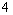Prof. Bryan Caplan

http://www.gmu.edu/departments/economics/bcaplan

Econ 345

Fall, 1998

Econometrics Pre-Test

This class requires competence in elementary mathematical statistics. You should definitely take the following pre-test to make sure that this is the right class for you. Answers for self-evaluation will be available next class.

Feel free to use books and calculators when taking the test, but spend no more than 90 minutes working on it.

Find E(X), E(X2), Var(X), and SD(X), where X is:

1. A random variable generated by rolling a normal six-sided die.

2. A random variable that is equal to 0 25% of the time, 1 50% of the time, 2 20% of the time, and 10 5% of the time.

3. A random variable generated by doubling a single roll of a normal six-sided die.

4. A random variable generated by summing two separate rolls of a normal six-sided die.

Evaluate the following expressions:

1.2.3.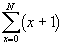4.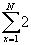Determine the Probability of the Following Events:

X and Y are two normal six-sided dice.

1. P(X-Y=0)

2. P(XY=40)

3. P(X2=X+Y)

4. P(X+Y<13)

X is a normal six-sided die; Y is a die with four sides numbered {1,2,3,4}.

1. P(X<5|X+Y=8)

2. P(X+Y>4|X<5)

3. P(XY<10|X+Y<8)

4. P(X/Y=1|X<5)

Take the Derivative of F(x):

1. F(x)=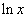2. F(x)=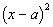3. F(x)=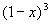4. F(x)=